# 深度学习利器：TensorFlow 与 NLP 模型

## 前言

2013 年末谷歌发布的 word2vec 工具，将一个词表示为词向量，将文字数字化，有效地应用于文本分析。2016 年谷歌开源自动生成文本摘要模型及相关 TensorFlow 代码。2016/2017 年，谷歌发布 / 升级语言处理框架 SyntaxNet，识别率提高 25%，为 40 种语言带来文本分割和词态分析功能。2017 年谷歌官方开源 tf-seq2seq，一种通用编码器 / 解码器框架，实现自动翻译。本文主要结合 TensorFlow 平台，讲解 TensorFlow 词向量生成模型（Vector Representations of Words）；使用 RNN、LSTM 模型进行语言预测；以及 TensorFlow 自动翻译模型。

## Word2Vec 数学原理简介

Word2Vec 中用到两个模型，CBOW 模型 (Continuous Bag-of-Words model) 和 Skip-gram 模型（Continuous Skip-gram Model）。模型示例如下，是三层结构的神经网络模型，包括输入层，投影层和输出层。

(点击放大图像)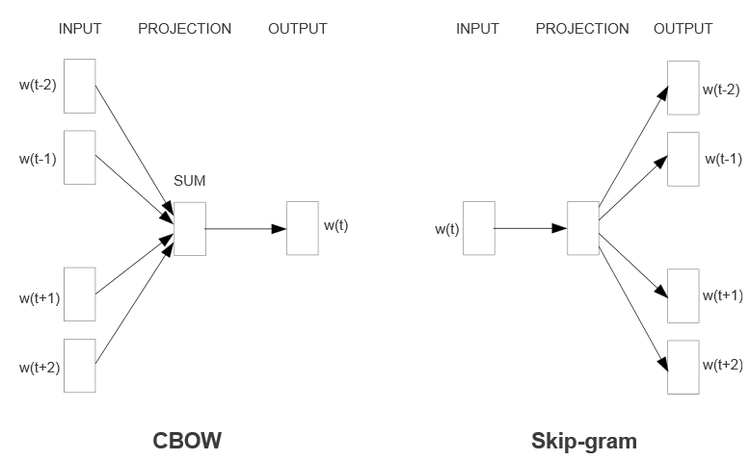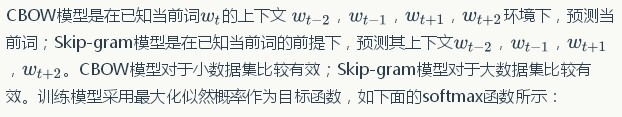(点击放大图像)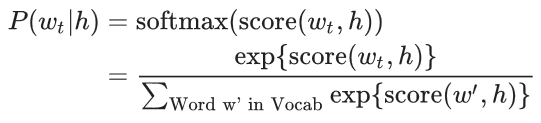(点击放大图像)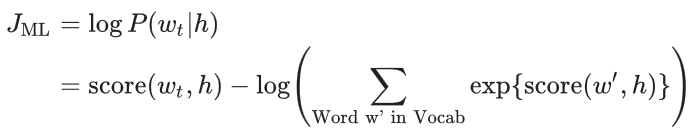(点击放大图像)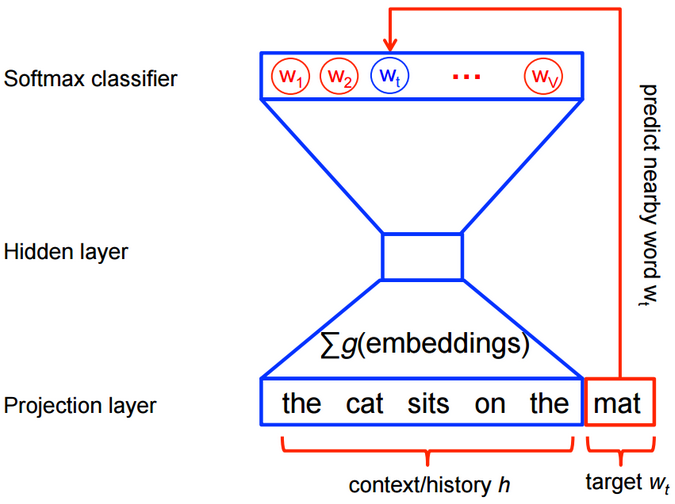(点击放大图像)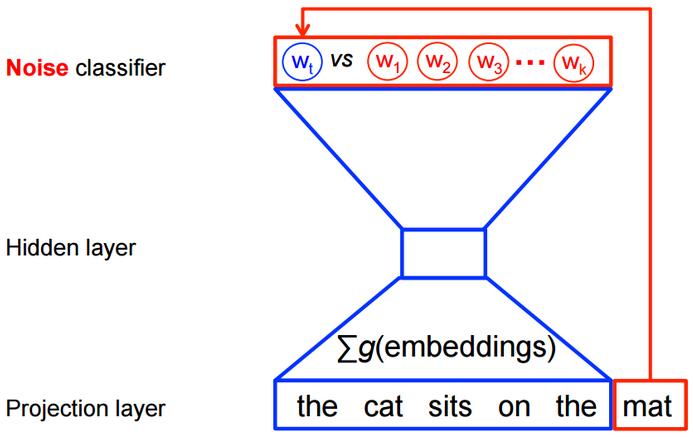## TensorFlow 近义词模型

(点击放大图像)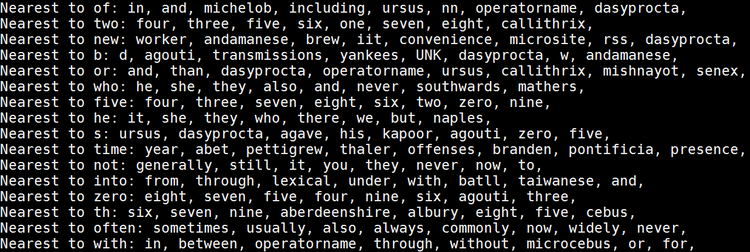## 构建词向量变量，vocabulary_size 为字典大小，embedding_size 为词向量大小

embeddings = tf.Variable(tf.random_uniform([vocabulary_size, embedding_size], -1.0, 1.0))

## 定义负采样中逻辑回归的权重和偏置

nce_weights = tf.Variable(tf.truncated_normal
([vocabulary_size, embedding_size], stddev=1.0 / math.sqrt(embedding_size)))
nce_biases = tf.Variable(tf.zeros([vocabulary_size]))

## 定义训练数据的接入

train_inputs = tf.placeholder(tf.int32, shape=[batch_size])
train_labels = tf.placeholder(tf.int32, shape=[batch_size, 1])

## 定义根据训练数据输入，并寻找对应的词向量

embed = tf.nn.embedding_lookup(embeddings, train_inputs)

## 基于负采样方法计算 Loss 值

loss = tf.reduce_mean( tf.nn.nce_loss
(weights=nce_weights, biases=nce_biases, labels=train_labels,
inputs=embed, num_sampled=num_sampled, num_classes=vocabulary_size))

## 定义使用随机梯度下降法执行优化操作，最小化 loss 值

optimizer = tf.train.GradientDescentOptimizer(learning_rate=1.0).minimize(loss)

## 通过 TensorFlow Session Run 的方法执行模型训练

for inputs, labels in generate_batch(...):
feed_dict = {train_inputs: inputs, train_labels: labels}
_, cur_loss = session.run([optimizer, loss], feed_dict=feed_dict)

## TensorFlow 语言预测模型

(点击放大图像)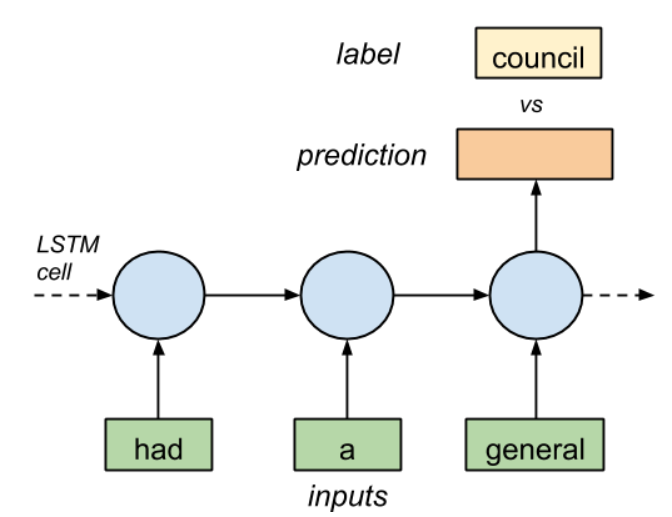### RNN 技术原理

(点击放大图像)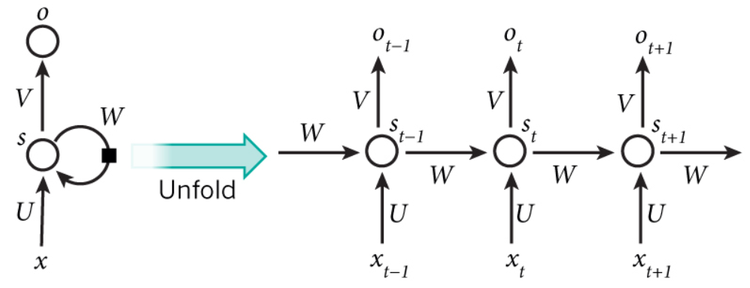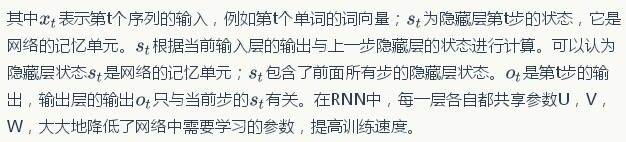### LSTM 技术原理

RNN 有一问题，反向传播时，梯度也会呈指数倍数的衰减，导致经过许多阶段传播后的梯度倾向于消失，不能处理长期依赖的问题。虽然 RNN 理论上可以处理任意长度的序列，但实习应用中，RNN 很难处理长度超过 10 的序列。为了解决 RNN 梯度消失的问题，提出了 Long Short-Term Memory 模块，通过门的开关实现序列上的记忆功能，当误差从输出层反向传播回来时，可以使用模块的记忆元记下来。所以 LSTM 可以记住比较长时间内的信息。常见的 LSTM 模块如下图所示：

(点击放大图像)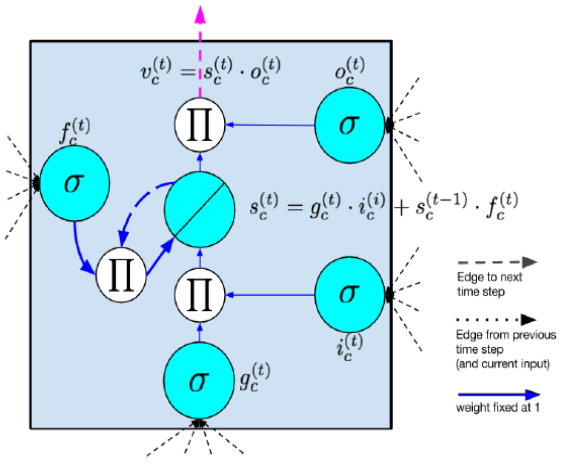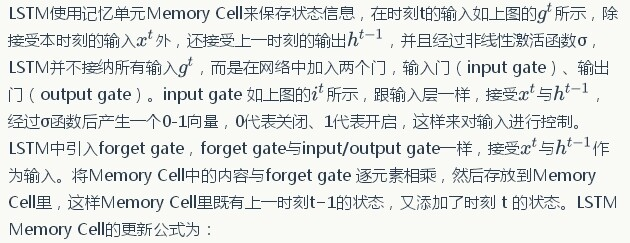(点击放大图像)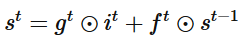output gate 类似于 input gate 同样会产生一个 0-1 向量来控制 Memory Cell 到输出层的输出，如下公式所示：

(点击放大图像)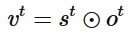## 读取训练数据

data = _read_words(filename)
#按照单词出现频率，进行排序
counter = collections.Counter(data)
count_pairs = sorted(counter.items(), key=lambda x: (-x1, x))
#构建词典及词典索引
words, _ = list(zip(*count_pairs))
word_to_id = dict(zip(words, range(len(words))))

## 读取训练数据单词，并转换为单词索引序列

data = _read_words(filename) data = [word_to_id[word] for word in data if word in word_to_id]

## 生成训练数据的 data 和 label，其中 epoch_size 为该 epoch 的训练迭代次数，num_steps 为 LSTM 的序列长度

i = tf.train.range_input_producer(epoch_size, shuffle=False).dequeue()
x = tf.strided_slice(data, [0, i * num_steps], [batch_size, (i + 1) * num_steps])
x.set_shape([batch_size, num_steps])
y = tf.strided_slice(data, [0, i * num_steps + 1], [batch_size, (i + 1) * num_steps + 1])
y.set_shape([batch_size, num_steps])

## 构建 LSTM Cell，其中 size 为隐藏神经元的数量

lstm_cell = tf.contrib.rnn.BasicLSTMCell(size,
forget_bias=0.0, state_is_tuple=True)

## 如果为训练模式，为保证训练鲁棒性，定义 dropout 操作

attn_cell = tf.contrib.rnn.DropoutWrapper(lstm_cell,
output_keep_prob=config.keep_prob)

## 根据层数配置，定义多层 RNN 神经网络

cell = tf.contrib.rnn.MultiRNNCell( [ attn_cell for _ in range(config.num_layers)],
state_is_tuple=True)

## 根据词典大小，定义词向量

embedding = tf.get_variable("embedding",
[vocab_size, size], dtype=data_type())

## 根据单词索引，查找词向量，如下图所示。从单词索引找到对应的 One-hot encoding，然后红色的 weight 就直接对应了输出节点的值，也就是对应的 embedding 向量。

inputs = tf.nn.embedding_lookup(embedding, input_.input_data)

(点击放大图像)## 定义 RNN 网络，其中 state 为 LSTM Cell 的状态，cell_output 为 LSTM Cell 的输出

for time_step in range(num_steps):
if time_step > 0: tf.get_variable_scope().reuse_variables()
(cell_output, state) = cell(inputs[:, time_step, :], state)
outputs.append(cell_output)

## 定义训练的 loss 值就，如下公式所示。

(点击放大图像)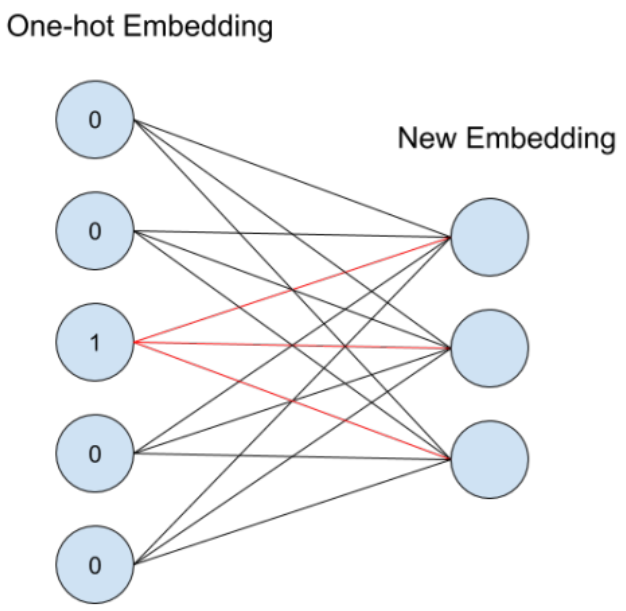softmax_w = tf.get_variable("softmax_w", [size, vocab_size], dtype=data_type())
softmax_b = tf.get_variable("softmax_b", [vocab_size], dtype=data_type())
logits = tf.matmul(output, softmax_w) + softmax_b

## Loss 值

loss = tf.contrib.legacy_seq2seq.sequence_loss_by_example([logits],
[tf.reshape(input_.targets, [-1])], [tf.ones([batch_size * num_steps], dtype=data_type())])

## 定义梯度及优化操作

cost = tf.reduce_sum(loss) / batch_size
tvars = tf.trainable_variables()
grads, _ = tf.clip_by_global_norm(tf.gradients(cost, tvars), config.max_grad_norm)
optimizer = tf.train.GradientDescentOptimizer(self._lr)

## 单词困惑度 eloss

perplexity = np.exp(costs / iters)

## TensorFlow 语言翻译模型

(点击放大图像)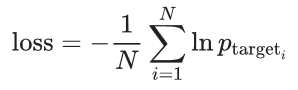(点击放大图像)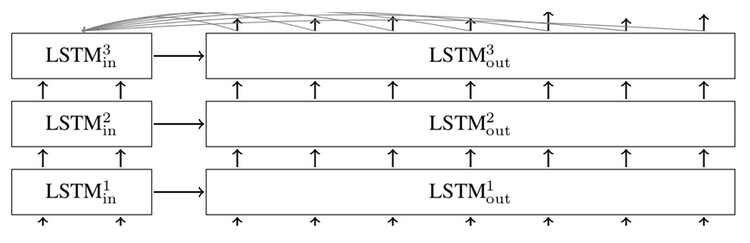• basic_rnn_seq2seq：输入和输出都是 embedding 的形式；encoder 和 decoder 用相同的 RNN cell，但不共享权值参数；

• tied_rnn_seq2seq：同 basic_rnn_seq2seq，但 encoder 和 decoder 共享权值参数；

• embedding_rnn_seq2seq：同 basic_rnn_seq2seq，但输入和输出改为 id 的形式，函数会在内部创建分别用于 encoder 和 decoder 的 embedding 矩阵；

• embedding_tied_rnn_seq2seq：同 tied_rnn_seq2seq，但输入和输出改为 id 形式，函数会在内部创建分别用于 encoder 和 decoder 的 embedding 矩阵；

• embedding_attention_seq2seq：同 embedding_rnn_seq2seq，但多了 attention 机制；

embedding_rnn_seq2seq 函数接口使用说明如下：

• encoder_inputs：encoder 的输入

• decoder_inputs：decoder 的输入

• cell：RNN_Cell 的实例

• num_encoder_symbols，num_decoder_symbols：分别是编码和解码的大小

• embedding_size：词向量的维度

• output_projection：decoder 的 output 向量投影到词表空间时，用到的投影矩阵和偏置项

• feed_previous：若为 True, 只有第一个 decoder 的输入符号有用，所有的 decoder 输入都依赖于上一步的输出；

outputs, states = embedding_rnn_seq2seq(
encoder_inputs, decoder_inputs, cell,
num_encoder_symbols, num_decoder_symbols,
embedding_size, output_projection=None,
feed_previous=False)

TensorFlow 官方提供了英语到法语的翻译示例，采用的是 statmt 网站提供的语料数据，主要包含：giga-fren.release2.fixed.en（英文语料，3.6G）和 giga-fren.release2.fixed.fr（法文语料，4.3G）。该示例的代码结构如下所示：

• seq2seq_model.py：seq2seq 的 TensorFlow 模型

采用了 embedding_attention_seq2seq 用于创建 seq2seq 模型。

• data_utils.py：对语料数据进行数据预处理，根据语料数据生成词典库；并基于词典库把要翻译的语句转换成用用词 ID 表示的训练序列。如下图所示：

(点击放大图像)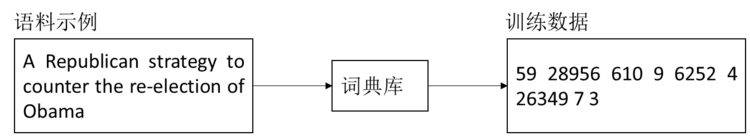• translate.py：主函数入口，执行翻译模型的训练

## 执行模型训练

python translate.py
--data_dir [your_data_directory] --train_dir [checkpoints_directory]
--en_vocab_size=40000 --fr_vocab_size=40000


## 参考文献

1. http://www.tensorflow.org
2. 深度学习利器: 分布式 TensorFlow 及实例分析
3. 深度学习利器：TensorFlow 使用实战
4. 深度学习利器：TensorFlow 系统架构与高性能程序设计
5. 深度学习利器：TensorFlow 与深度卷积神经网络

## 作者简介